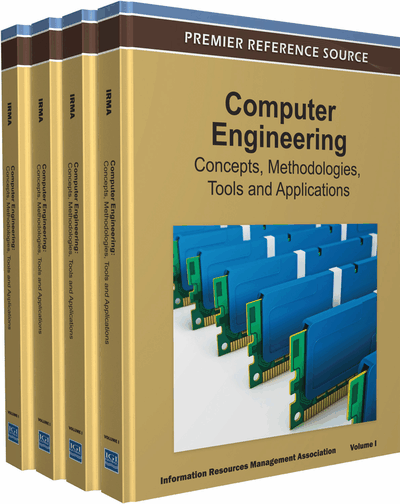# Macroeconomic Forecasting Using Genetic Programming Based Vector Error Correction Model

Xi Chen (Deloitte Financial Advisory Services, China), Ye Pang (The People’s Insurance Company (Group) of China Limited, China) and Guihuan Zheng (The People’s Bank of China, China)
DOI: 10.4018/978-1-61350-456-7.ch317

## Abstract

Vector autoregressions are widely used in macroeconomic forecasting since they became known in the 1970s. Extensions including vector error correction models, co-integration and dynamic factor models are all rooted in the framework of vector autoregression. The three important extensions are demonstrated to have formal equivalence between each other. Above all, they all emphasize the importance of “common trends” or “common factors”. Many researches, including a series of work of Stock and Watson, find that “common factor” models significantly improve accuracy in forecasting macroeconomic time series. This study follows the work of Stock and Watson. The authors propose a hybrid framework called genetic programming based vector error correction model (GPVECM), introducing genetic programming to traditional econometric models. This new method could construct common factors directly from nonstationary data set, avoiding differencing the original data and thus preserving more information. The authors’ model guarantees that the constructed common factors satisfy the requirements of econometric models such as co-integration, in contrast to the traditional approach. Finally but not trivially, their model could save lots of time and energy from repeated work of unit root tests and differencing, which they believe is convenient for practitioners. An empirical study of forecasting US import from China is reported. The results of the new method dominates those of the plain vector error correction model and the ARIMA model.
Chapter Preview
Top

## 1. Introduction

Macroeconomic forecasting is of great importance in practice. It helps governments and companies perceive the future of the economy and make right decisions.

### 1.1 Overview of the Development of Macroeconomic Forecasting

There are two important approaches to forecast macroeconomic indicators. One is the structural approach, the other is the reduced form approach. By the late 1970s, Keynesian structural macroeconomic forecasting was receding. One response is to augment the traditional system-of-equations econometrics in attempts to remedy its defects. Another response was to explore nonstructural forecasting methods. The title of an important paper by Sargent and Sims (1977), “Business Cycle Modeling Without Pretending to Have Too Much a Priori Theory” nicely summarizes the spirit of the times.

Back to the 1920s, Slutsky (1927) and Yule (1927) argued that simple linear difference equations provide a powerful framework for economic and financial forecasting. Such stochastic difference equations are called autoregressions. In the 1930s, mathematicians Wiener, Kolmogorov and Wold worked out the fomulae for optimal forecasts from models by Slutsky and Yule.

In the 1970s, Box and Jenkins published their celebrated book on time series analysis and forecasting, another milestone in the development of non-structural forecasting. Since then, researches inspired by Box and Jenkins grew explosively. Box and Jenkins allowed trend to be driven by the cumulative effects of random shocks, resulting in “stochastic trend”. Stock and Watson (1988a) provide an insightful discussion of stochastic tread and its wide-ranging implications. Autoregressive moving average (ARMA) models are the centerpiece of the Box-Jenkins framework. ARMA models are combinations of the autoregressive and moving average models of Slutsky and Yule, and they have more parsimonious forms.

The work followed Box and Jenkins is focused on cross-variable relationship, whereas the basic Box-Jenkins models are univariate. Thus, vector autoregressive models have emerged as the central multivariate model. Vector autoregressions are less restrictive than system-of-equations models, since all variables are endogenous rather than arbitrarily labeled “endogenous” or “exogenous”.

In a vector autoregression, each of a set of variables is regressed on past values of itself and past values of every other variable in the system. Cross-variable linkages are automatically incorporated because lags of all variables are included in each equation, and because error terms are allowed to be correlated among equations. However it turns out that vector autoregressions could be estimated exactly by OLS equationwise despite the correlation of the error terms.

Many multivariate extensions of the Box-Jenkins paradigm are implemented in the vector autoregressive framework. One important contribution is the dynamic factor model of Sargent and Sims (1977) and Geweke (1977). The essential idea of dynamic factor models is that some economic shocks are common across sectors and others are idiosyncratic, so that large sets of variables may depend heavily on just a few common underlying sources of variation. Dynamic factor models are particularly useful with the emergence of long horizon data set.

Granger (1981) and Engle and Granger (1987) developed the idea of cointegration. Two series are cointegrated if each contains a stochastic trends, yet (one of) their linear combination does not. The idea of the shared stochastic trend is consistent with the idea of common factor. This is the essence of the Stock and Watson (1988b) “common trends” representation of cointegrated systems and is precisely the same idea as with the dynamic factor model: comovements may be due to dependence on common factors. Cointegration is also closely connected to the idea of error-correction, pioneered by Sargan (1964). Indeed there is a formal equivalence between cointegration and error correction, as established by Engle and Granger (1987).

Our work follows the “common trend” approach. Stock and Watson (2002) demonstrated that the latent common factors could be precisely estimated by principal component analysis when the number of variables and time series observations are large enough. Stock and Watson (2002) focused on macroeconomic forecasting using common factors based on principal components. They adopt a two-step procedure: first, the latent factors are estimated by principal component analysis; second, the relation between the regressand and the latent common factors is estimated by linear regression. Stock and Watson (2005) compared several models and concluded that factor models produce the best forecasting accuracy.

## Complete Chapter List

Search this Book:
Reset